# RS Aggarwal Solutions for Class 10 Maths Chapter 3 Linear Equations in Two Variables Exercise 3B

R S Aggarwal Solutions for Class 10 Maths Chapter 3 Linear Equations in Two Variables, contains solutions for exercise 3B questions. Exercise 3b is all about solving simultaneous linear equations using algebraic methods. Students can download the R S Aggarwal Solutions of Class 10  maths and clear their doubts on the concept.

## Download PDF of RS Aggarwal Solutions for Class 10 Maths Chapter 3 Linear Equations in Two Variables Exercise 3B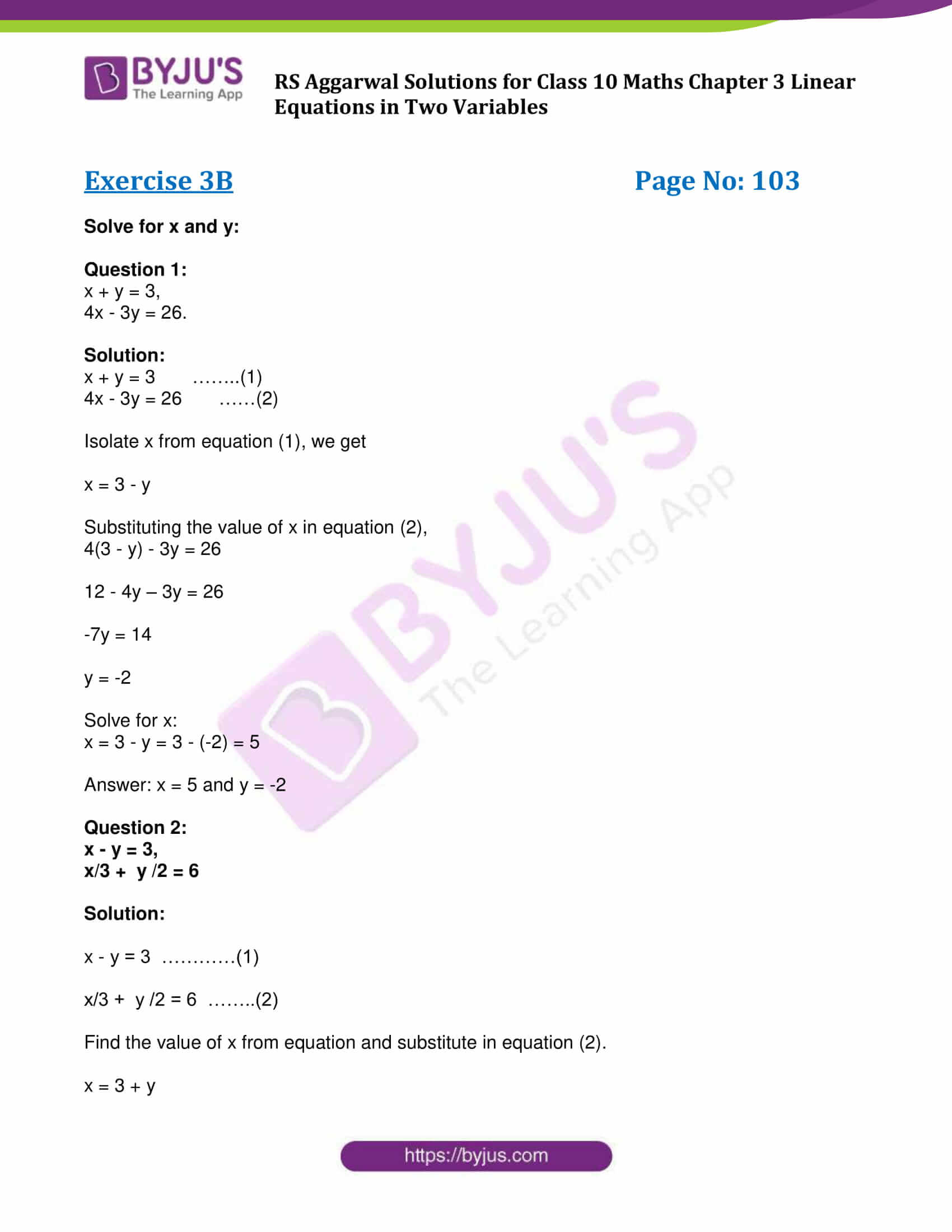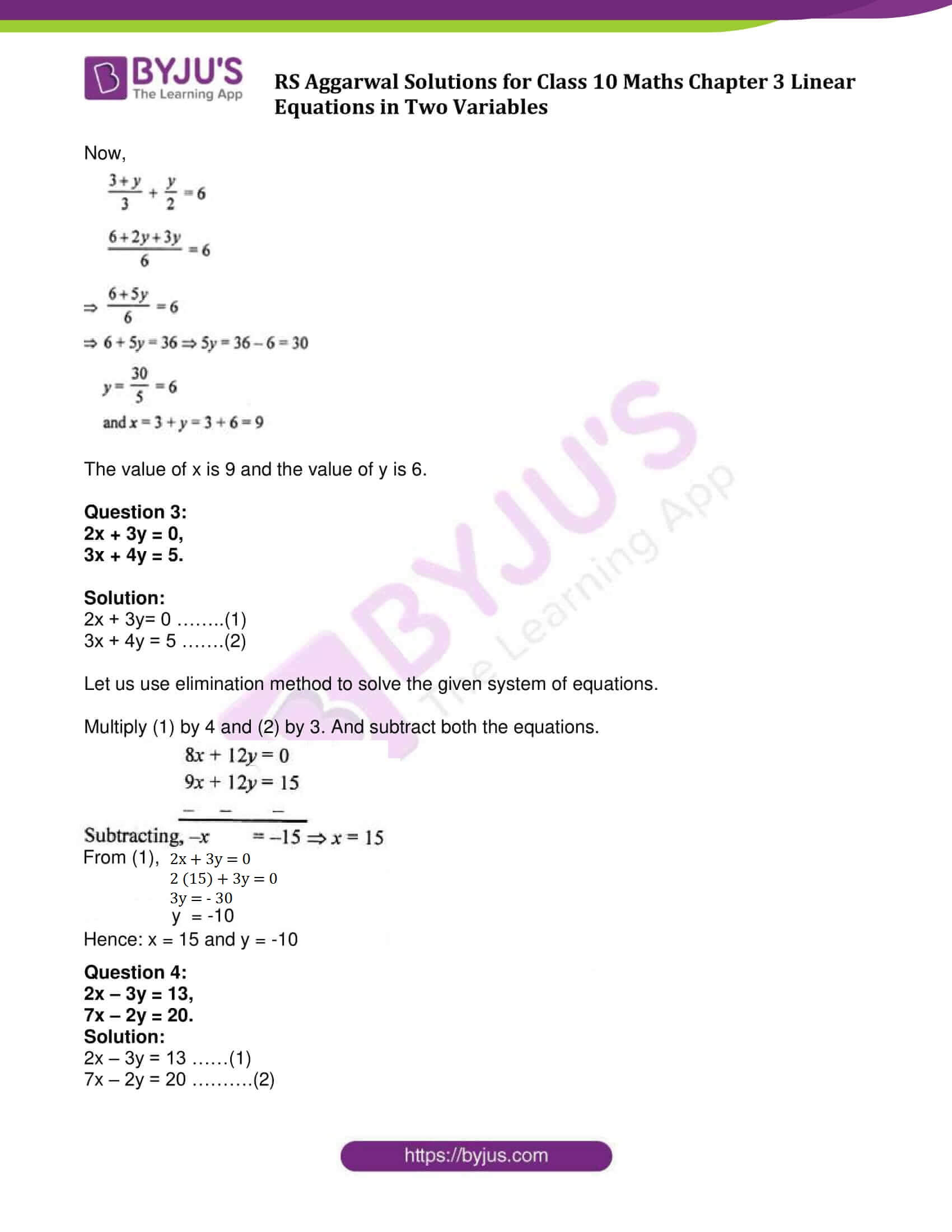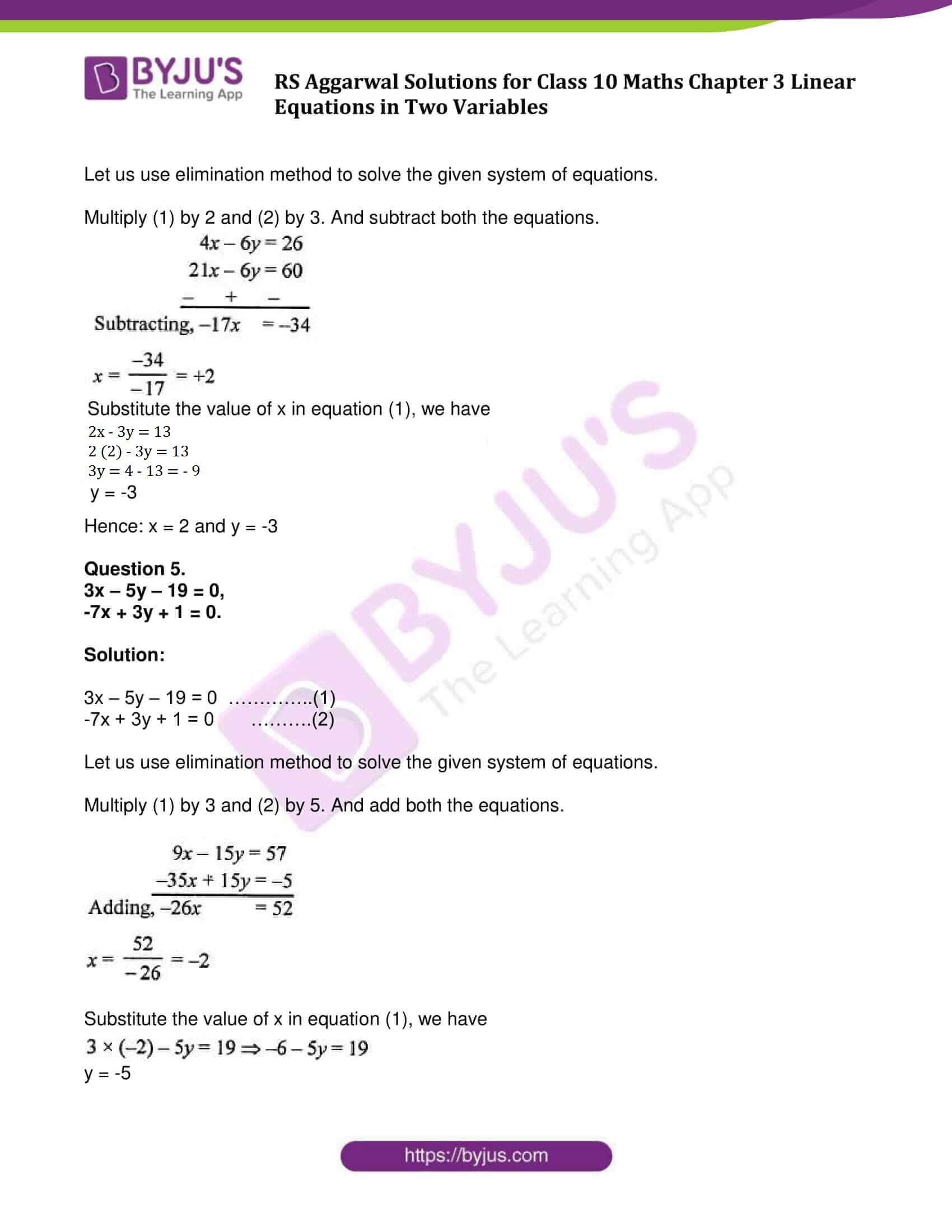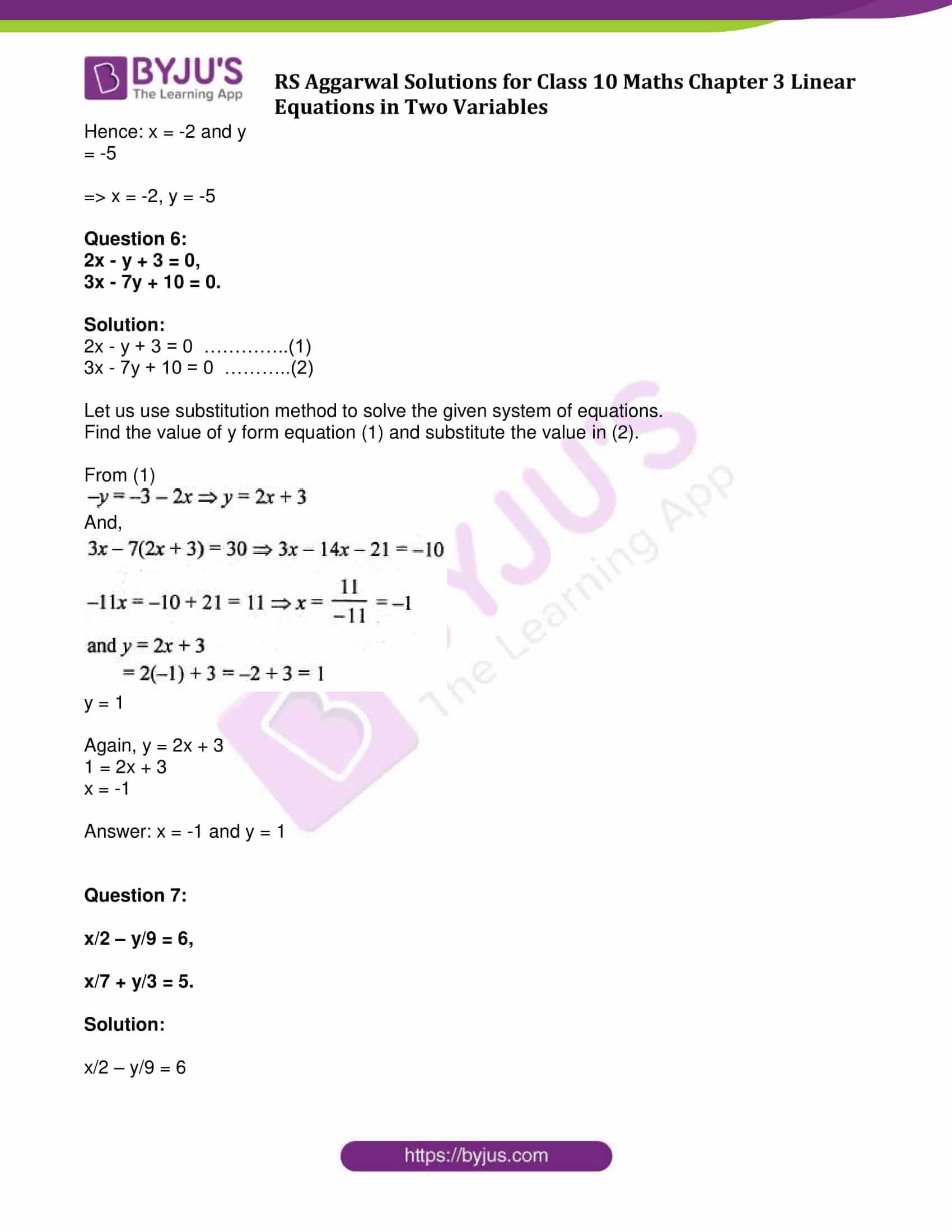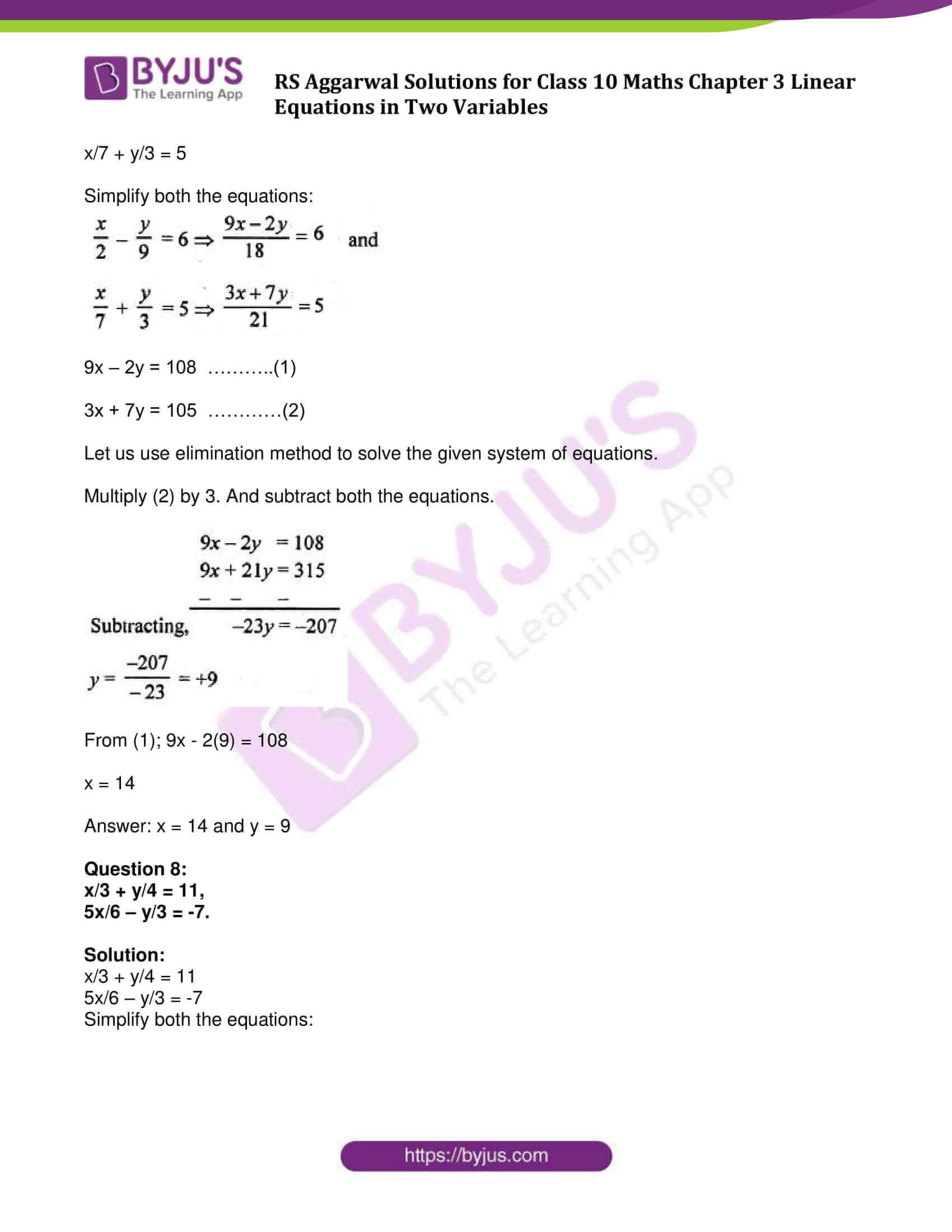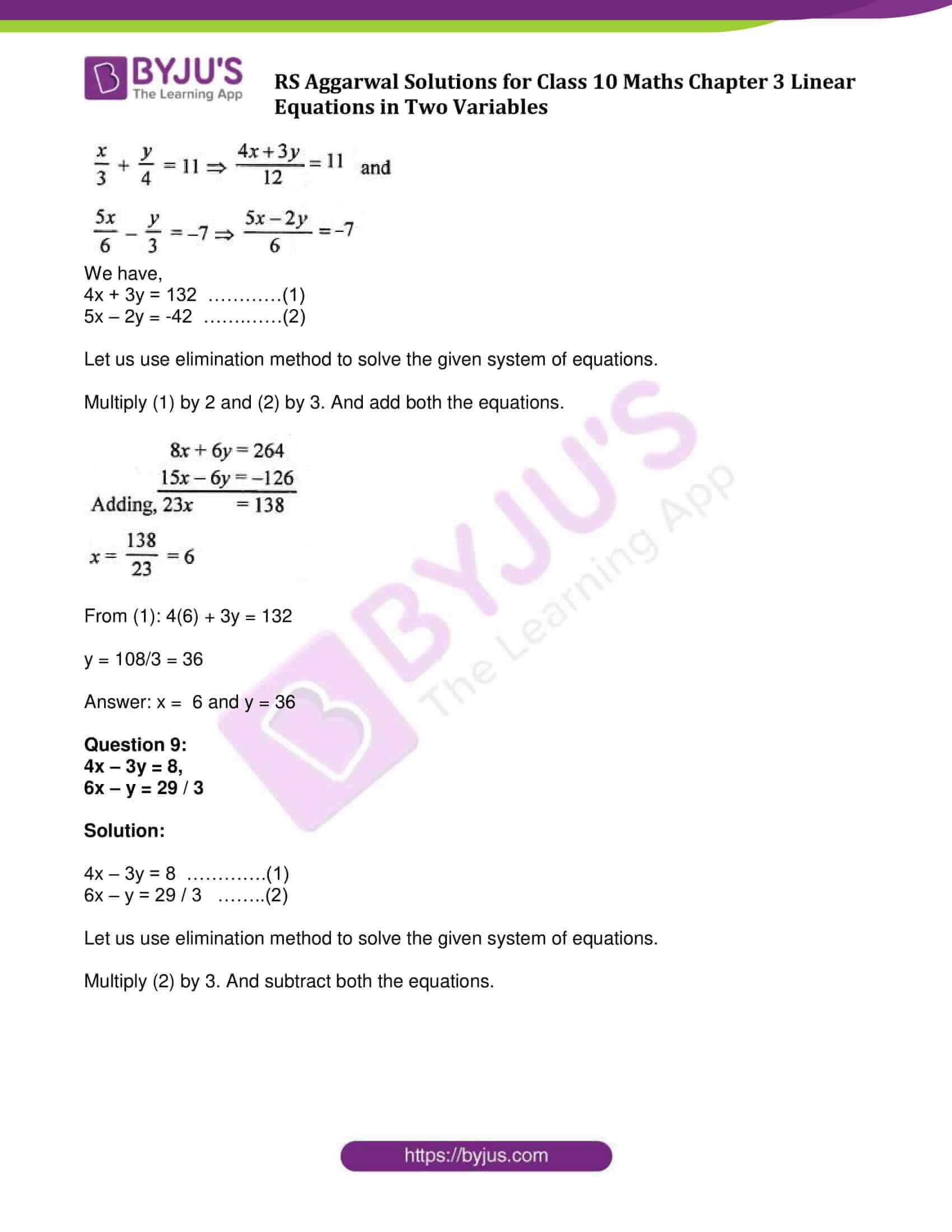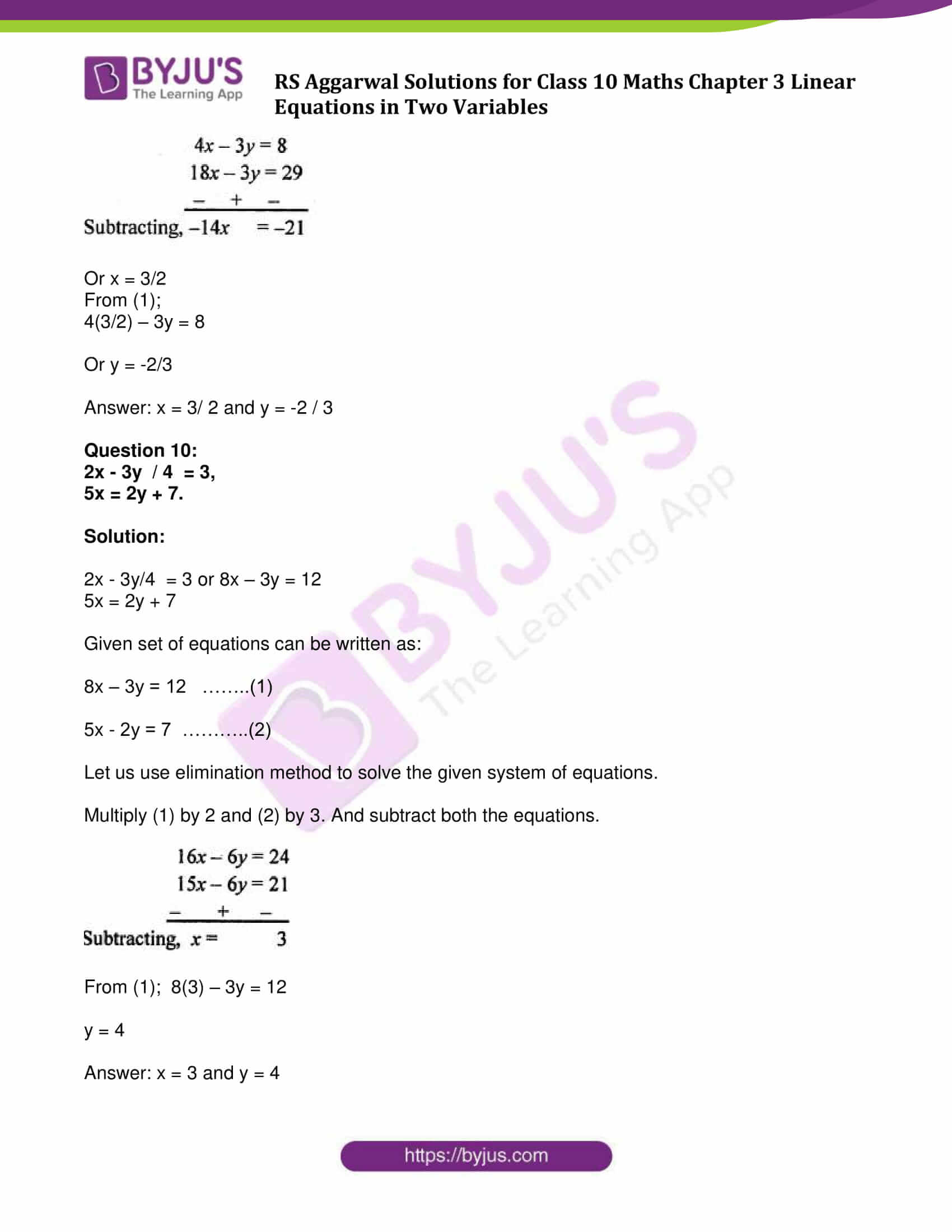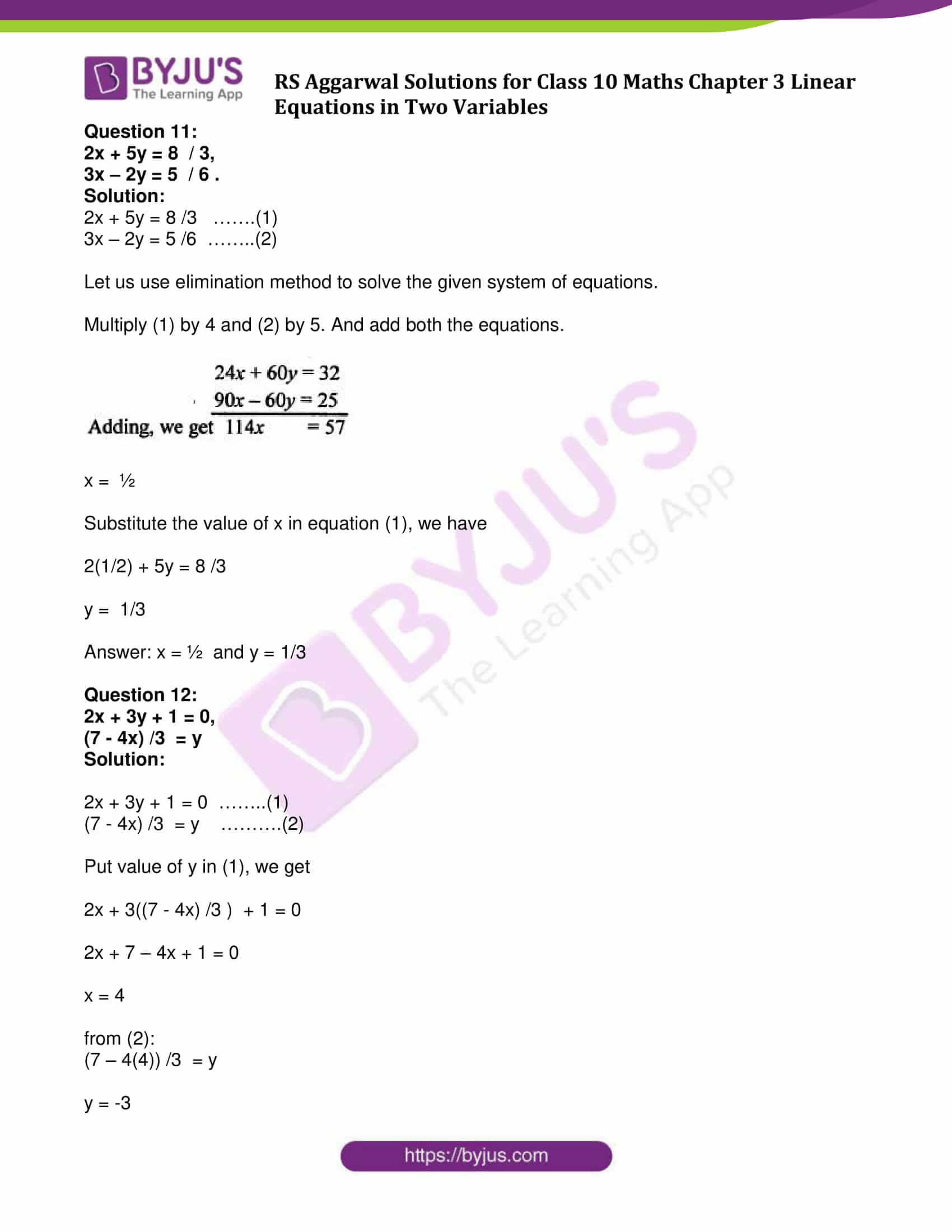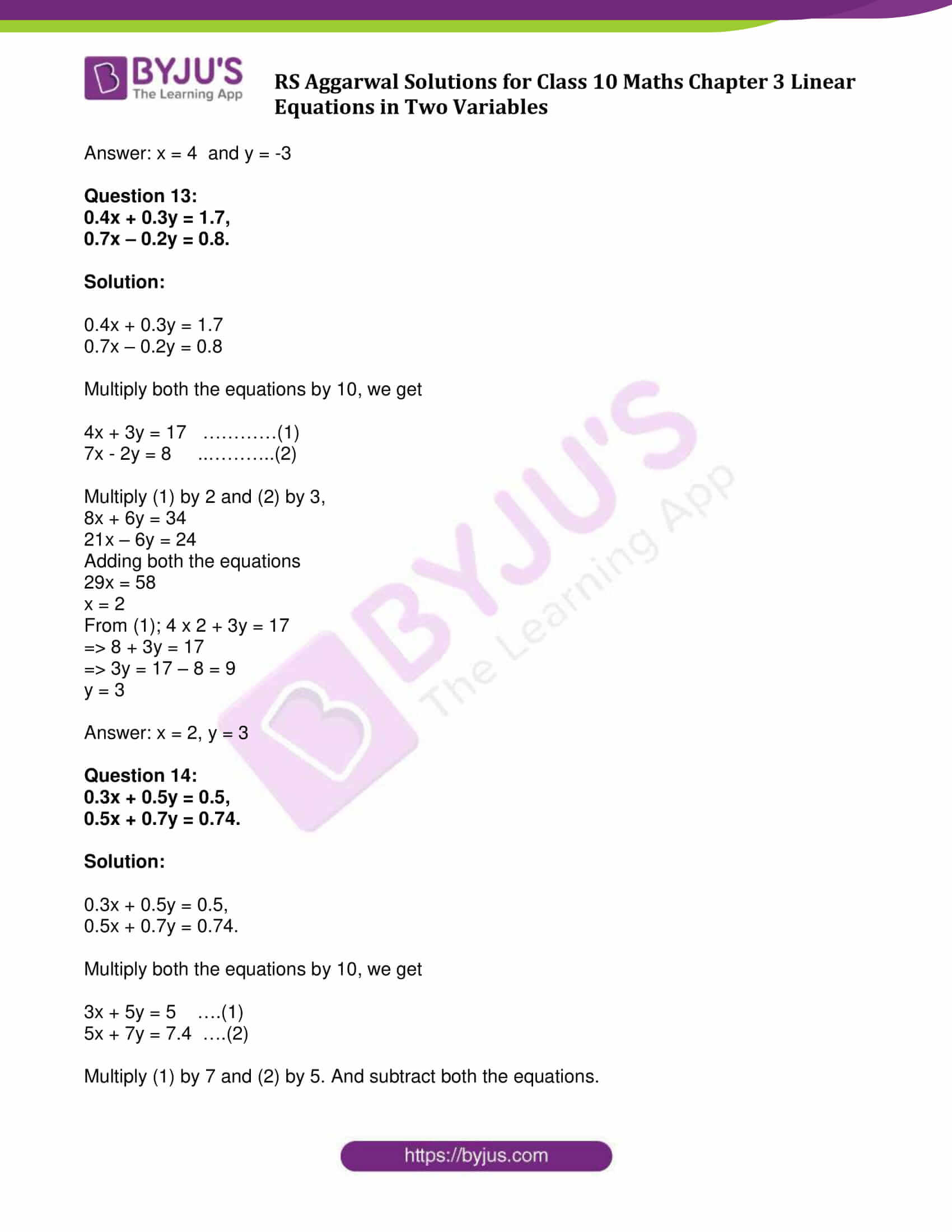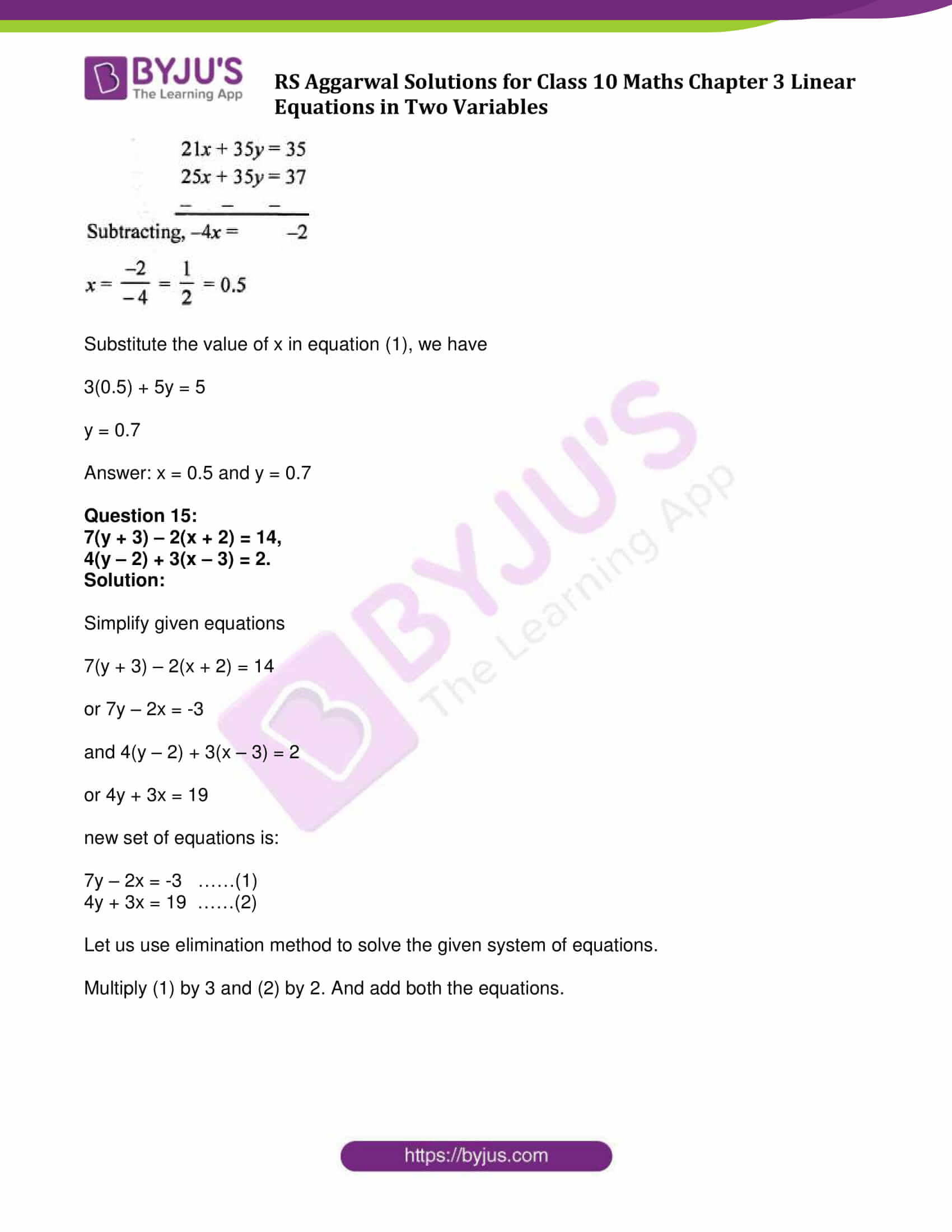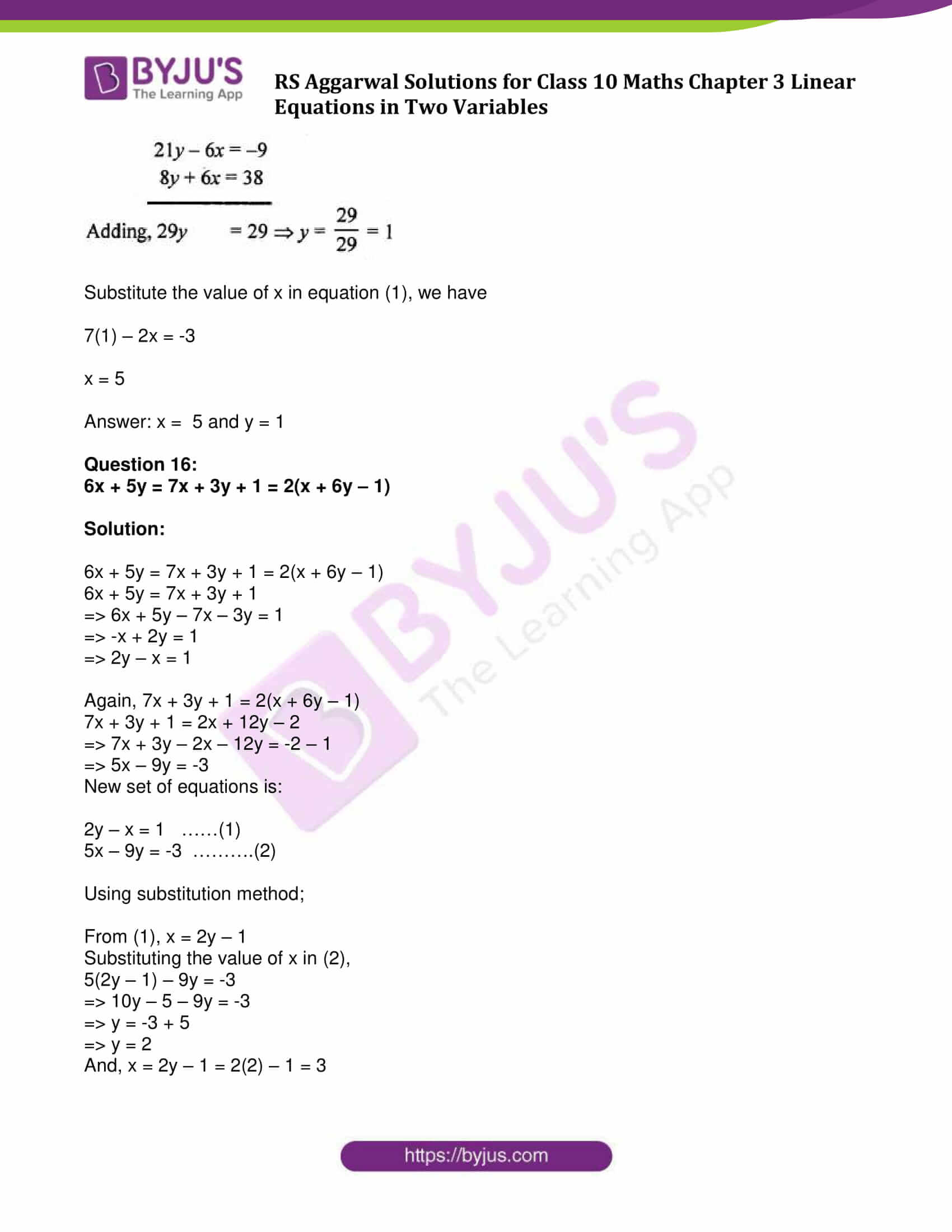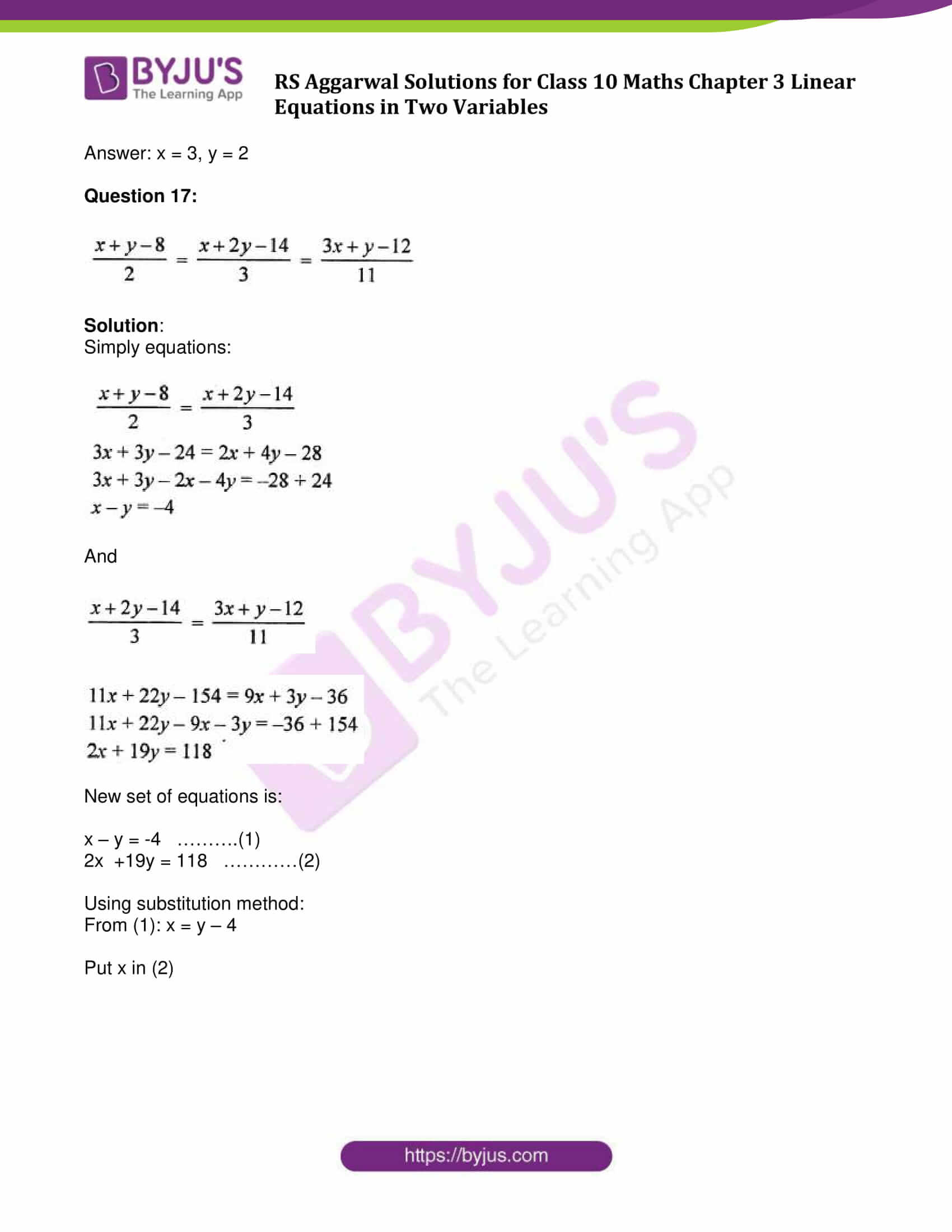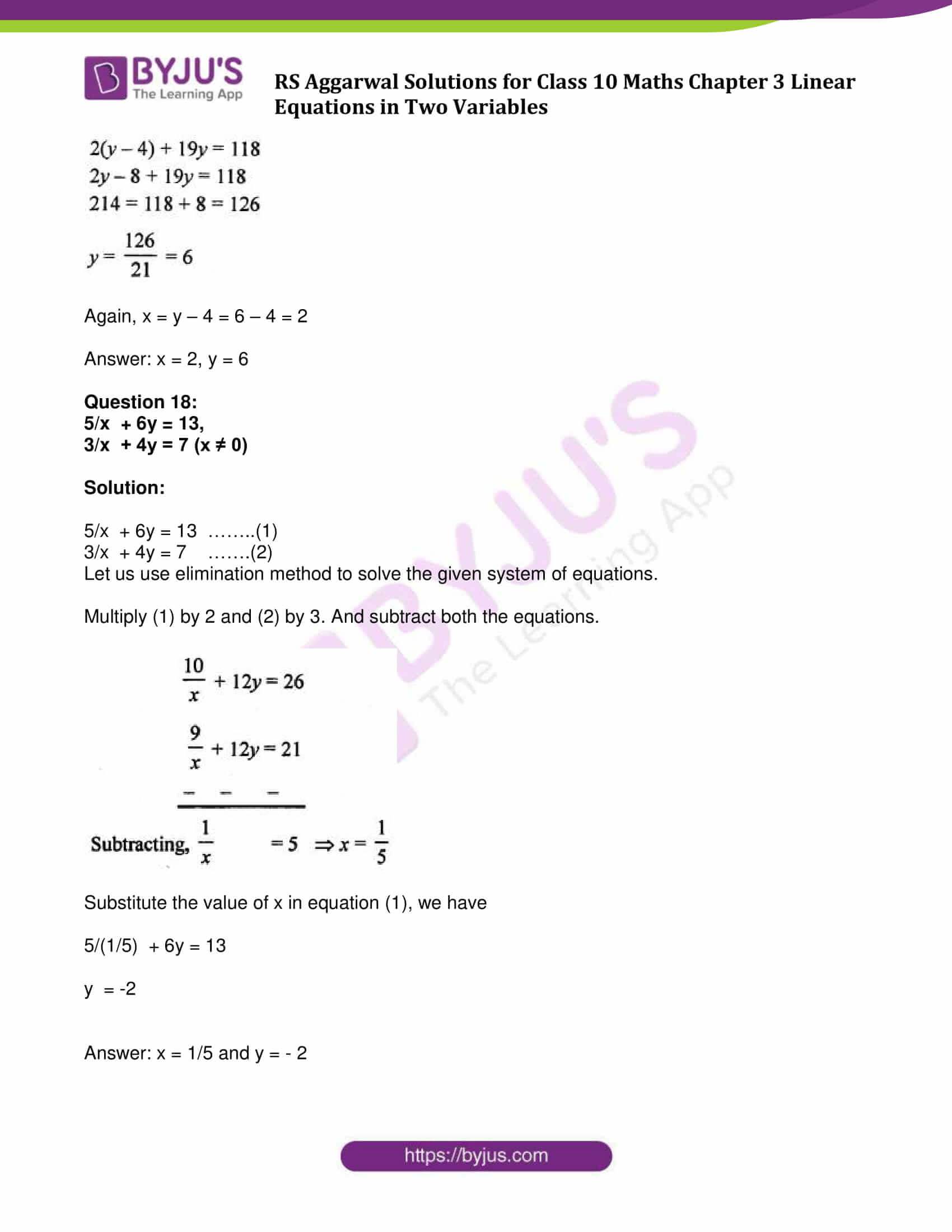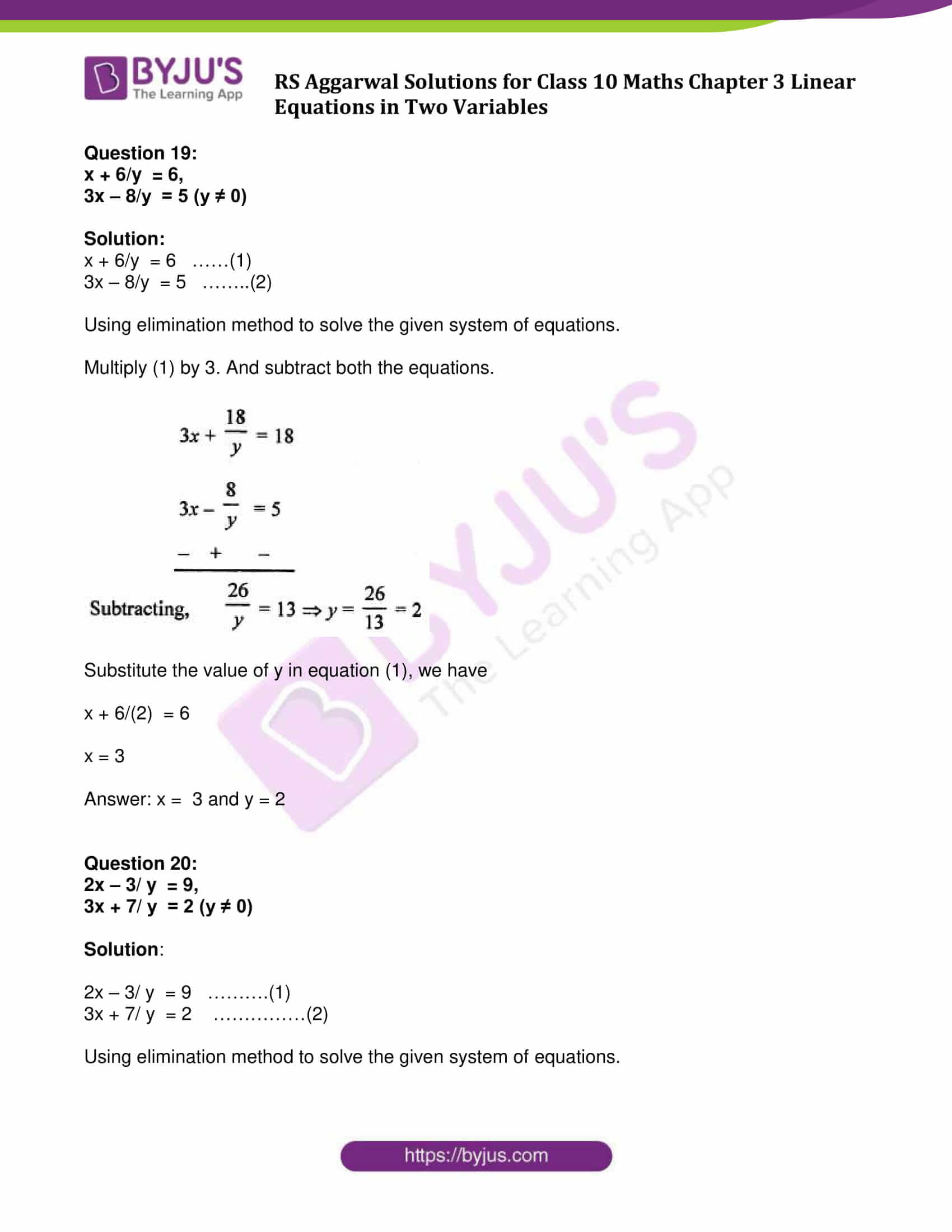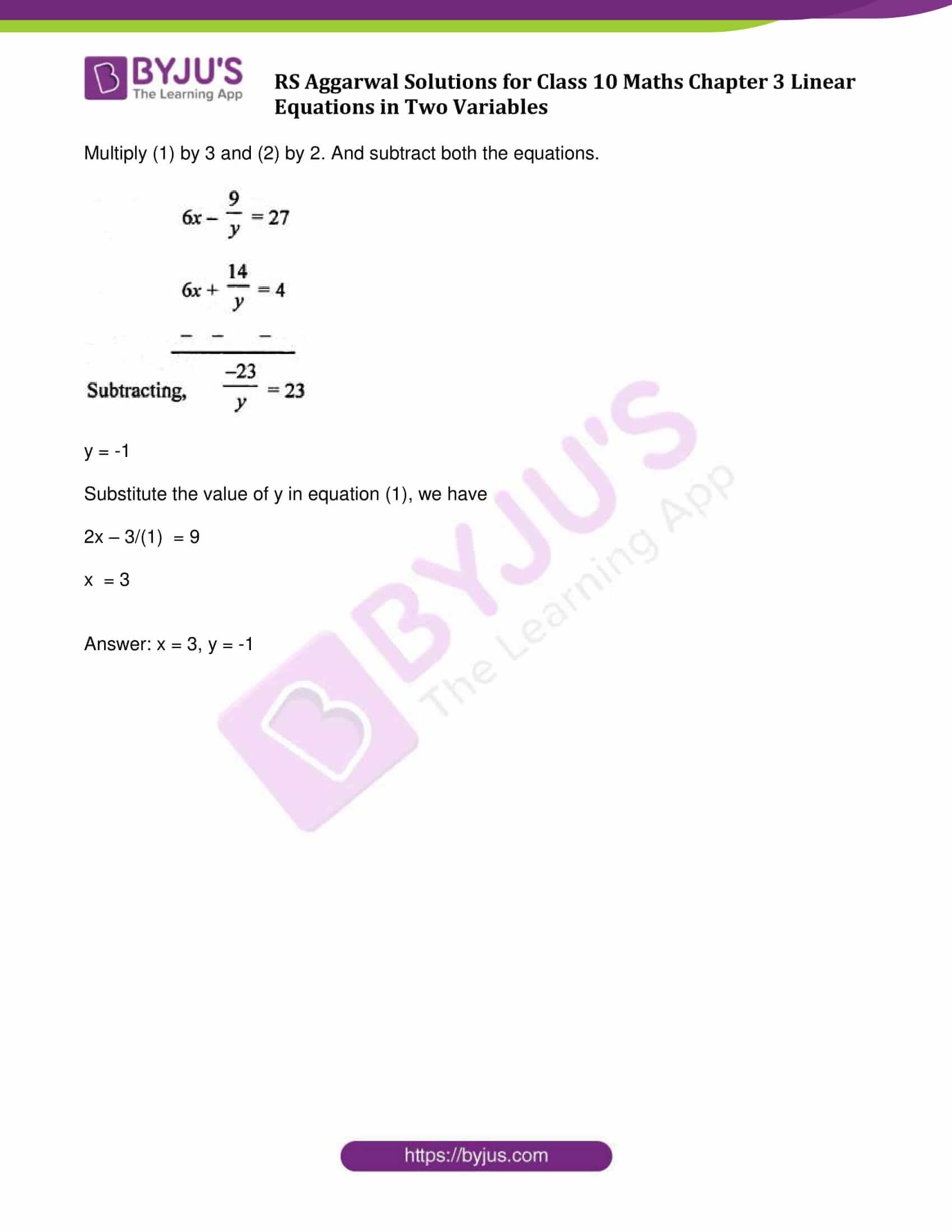### Access other exercise solutions of Class 10 Maths Chapter 3 Linear Equations in Two Variables

Exercise 3A Solutions: 29 Questions (Long Answers)

Exercise 3C Solutions: 13 Questions (Long Answers)

Exercise 3D Solutions: 14 Questions (Short Answers)

Exercise 3E Solutions: 20 Questions (Long Answers)

Exercise 3F Solutions: 10 Questions (6 Short Answers and 4 Long Answers)

## Exercise 3B Page No: 103

Solve for x and y:

Question 1:

x + y = 3,

4x – 3y = 26.

Solution:

x + y = 3 ……..(1)

4x – 3y = 26 ……(2)

Isolate x from equation (1), we get

x = 3 – y

Substituting the value of x in equation (2),

4(3 – y) – 3y = 26

12 – 4y – 3y = 26

-7y = 14

y = -2

Solve for x:

x = 3 – y = 3 – (-2) = 5

Answer: x = 5 and y = -2

Question 2:

x – y = 3,

x/3 + y /2 = 6

Solution:

x – y = 3 …………(1)

x/3 + y /2 = 6 ……..(2)

Find the value of x from equation and substitute in equation (2).

x = 3 + y

Now,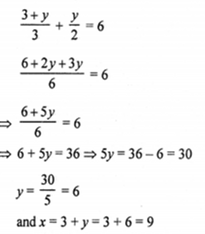The value of x is 9 and the value of y is 6.

Question 3:

2x + 3y = 0,

3x + 4y = 5.

Solution:

2x + 3y= 0 ……..(1)

3x + 4y = 5 …….(2)

Let us use elimination method to solve the given system of equations.

Multiply (1) by 4 and (2) by 3. And subtract both the equations.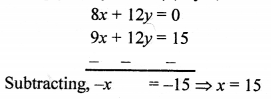From (1),

2x + 3y = 0

2 (15) + 3y = 0

3y = – 30

y = -10

Hence: x = 15 and y = -10

Question 4:

2x – 3y = 13,

7x – 2y = 20.

Solution:

2x – 3y = 13 ……(1)

7x – 2y = 20 ……….(2)

Let us use elimination method to solve the given system of equations.

Multiply (1) by 2 and (2) by 3. And subtract both the equations.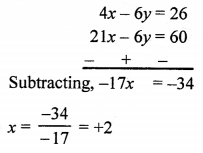Substitute the value of x in equation (1), we have

2x – 3y = 13

2 (2) – 3y = 13

3y = 4 – 13 = – 9

y = -3

Hence: x = 2 and y = -3

Question 5.

3x – 5y – 19 = 0,

-7x + 3y + 1 = 0.

Solution:

3x – 5y – 19 = 0 …………..(1)

-7x + 3y + 1 = 0 ……….(2)

Let us use elimination method to solve the given system of equations.

Multiply (1) by 3 and (2) by 5. And add both the equations.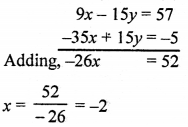Substitute the value of x in equation (1), we have

3 × (-2) -5y = 19 &#8680; -6 -5y = 19

y = -5

Hence: x = -2 and y = -5

⇨ x = -2, y = -5

Question 6:

2x – y + 3 = 0,

3x – 7y + 10 = 0.

Solution:

2x – y + 3 = 0 …………..(1)

3x – 7y + 10 = 0 ………..(2)

Let us use substitution method to solve the given system of equations.

Find the value of y form equation (1) and substitute the value in (2).

From (1)

-y = -3 – 2x ⇨ y = 2x + 3

And,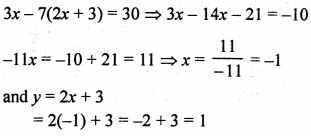y = 1

Again, y = 2x + 3

1 = 2x + 3

x = -1

Answer: x = -1 and y = 1

Question 7:

x/2 – y/9 = 6,

x/7 + y/3 = 5.

Solution:

x/2 – y/9 = 6

x/7 + y/3 = 5

Simplify both the equations: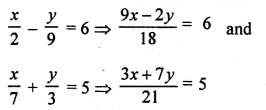9x – 2y = 108 ………..(1)

3x + 7y = 105 …………(2)

Let us use elimination method to solve the given system of equations.

Multiply (2) by 3. And subtract both the equations.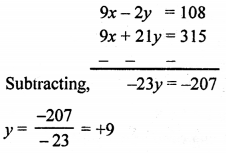From (1); 9x – 2(9) = 108

x = 14

Answer: x = 14 and y = 9

Question 8:

x/3 + y/4 = 11,

5x/6 – y/3 = -7.

Solution:

x/3 + y/4 = 11

5x/6 – y/3 = -7

Simplify both the equations: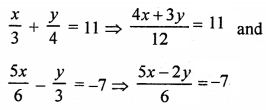We have,

4x + 3y = 132 …………(1)

5x – 2y = -42 …….……(2)

Let us use elimination method to solve the given system of equations.

Multiply (1) by 2 and (2) by 3. And add both the equations.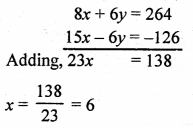From (1): 4(6) + 3y = 132

y = 108/3 = 36

Answer: x = 6 and y = 36

Question 9:

4x – 3y = 8,

6x – y = 29 / 3

Solution:

4x – 3y = 8 ………….(1)

6x – y = 29 / 3 ……..(2)

Let us use elimination method to solve the given system of equations.

Multiply (2) by 3. And subtract both the equations.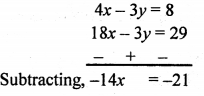Or x = 3/2

From (1);

4(3/2) – 3y = 8

Or y = -2/3

Answer: x = 3/ 2 and y = -2 / 3

Question 10:

2x – 3y / 4 = 3,

5x = 2y + 7.

Solution:

2x – 3y/4 = 3 or 8x – 3y = 12

5x = 2y + 7

Given set of equations can be written as:

8x – 3y = 12 ……..(1)

5x – 2y = 7 ………..(2)

Let us use elimination method to solve the given system of equations.

Multiply (1) by 2 and (2) by 3. And subtract both the equations.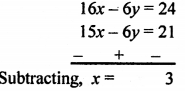From (1); 8(3) – 3y = 12

y = 4

Answer: x = 3 and y = 4

Question 11:

2x + 5y = 8 / 3,

3x – 2y = 5 / 6 .

Solution:

2x + 5y = 8 /3 …….(1)

3x – 2y = 5 /6 ……..(2)

Let us use elimination method to solve the given system of equations.

Multiply (1) by 4 and (2) by 5. And add both the equations.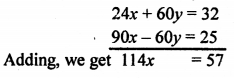x = ½

Substitute the value of x in equation (1), we have

2(1/2) + 5y = 8 /3

y = 1/3

Answer: x = ½ and y = 1/3

Question 12:

2x + 3y + 1 = 0,

(7 – 4x) /3 = y

Solution:

2x + 3y + 1 = 0 ……..(1)

(7 – 4x) /3 = y ……….(2)

Put value of y in (1), we get

2x + 3((7 – 4x) /3 ) + 1 = 0

2x + 7 – 4x + 1 = 0

x = 4

from (2):

(7 – 4(4)) /3 = y

y = -3

Answer: x = 4 and y = -3

Question 13:

0.4x + 0.3y = 1.7,

0.7x – 0.2y = 0.8.

Solution:

0.4x + 0.3y = 1.7

0.7x – 0.2y = 0.8

Multiply both the equations by 10, we get

4x + 3y = 17 …………(1)

7x – 2y = 8 ..………..(2)

Multiply (1) by 2 and (2) by 3,

8x + 6y = 34

21x – 6y = 24

29x = 58

x = 2

From (1); 4 x 2 + 3y = 17

⇨ 8 + 3y = 17

⇨ 3y = 17 – 8 = 9

y = 3

Answer: x = 2, y = 3

Question 14:

0.3x + 0.5y = 0.5,

0.5x + 0.7y = 0.74.

Solution:

0.3x + 0.5y = 0.5,

0.5x + 0.7y = 0.74.

Multiply both the equations by 10, we get

3x + 5y = 5 ….(1)

5x + 7y = 7.4 ….(2)

Multiply (1) by 7 and (2) by 5. And subtract both the equations.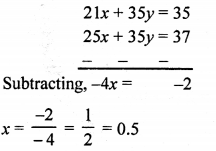Substitute the value of x in equation (1), we have

3(0.5) + 5y = 5

y = 0.7

Answer: x = 0.5 and y = 0.7

Question 15:

7(y + 3) – 2(x + 2) = 14,

4(y – 2) + 3(x – 3) = 2.

Solution:

Simplify given equations

7(y + 3) – 2(x + 2) = 14

or 7y – 2x = -3

and 4(y – 2) + 3(x – 3) = 2

or 4y + 3x = 19

new set of equations is:

7y – 2x = -3 ……(1)

4y + 3x = 19 ……(2)

Let us use elimination method to solve the given system of equations.

Multiply (1) by 3 and (2) by 2. And add both the equations.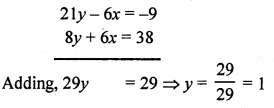Substitute the value of x in equation (1), we have

7(1) – 2x = -3

x = 5

Answer: x = 5 and y = 1

Question 16:

6x + 5y = 7x + 3y + 1 = 2(x + 6y – 1)

Solution:

6x + 5y = 7x + 3y + 1 = 2(x + 6y – 1)

6x + 5y = 7x + 3y + 1

⇨ 6x + 5y – 7x – 3y = 1

⇨ -x + 2y = 1

⇨ 2y – x = 1

Again, 7x + 3y + 1 = 2(x + 6y – 1)

7x + 3y + 1 = 2x + 12y – 2

⇨ 7x + 3y – 2x – 12y = -2 – 1

⇨ 5x – 9y = -3

New set of equations is:

2y – x = 1 ……(1)

5x – 9y = -3 ……….(2)

Using substitution method;

From (1), x = 2y – 1

Substituting the value of x in (2),

5(2y – 1) – 9y = -3

⇨ 10y – 5 – 9y = -3

⇨ y = -3 + 5

⇨ y = 2

And, x = 2y – 1 = 2(2) – 1 = 3

Answer: x = 3, y = 2

Question 17: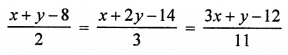Solution:

Simply equations: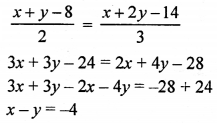And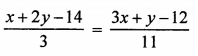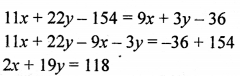New set of equations is:

x – y = -4 ……….(1)

2x +19y = 118 …………(2)

Using substitution method:

From (1): x = y – 4

Put x in (2)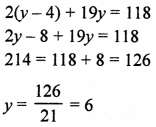Again, x = y – 4 = 6 – 4 = 2

Answer: x = 2, y = 6

Question 18:

5/x + 6y = 13,

3/x + 4y = 7 (x ≠ 0)

Solution:

5/x + 6y = 13 ……..(1)

3/x + 4y = 7 …….(2)

Let us use elimination method to solve the given system of equations.

Multiply (1) by 2 and (2) by 3. And subtract both the equations.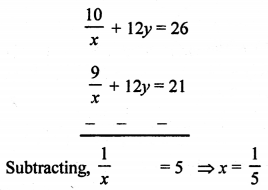Substitute the value of x in equation (1), we have

Answer: x = and y =

Question 19.

x + 6 / y = 6,

3x – 8 / y = 5 (y ≠ 0)

Solution:

Question 20.

2x – 3 / y = 9,

3x + 7 / y = 2 (y ≠ 0)

Solution:

x = 3, y = -1

## RS Aggarwal Solutions for Class 10 Maths Chapter 3 Linear Equations in Two Variables Exercise 3B

Class 10 Maths Chapter 3 Linear Equations in Two Variables Exercise 3B is based on the topic: Solving simultaneous linear equations using algebraic methods (which includes, substitution method and elimination method).